# Solving Equations of Motion for Systems of Particles with MATLAB (Part - 4) Civil Engineering (CE) Notes | EduRev

## Civil Engineering (CE) : Solving Equations of Motion for Systems of Particles with MATLAB (Part - 4) Civil Engineering (CE) Notes | EduRev

The document Solving Equations of Motion for Systems of Particles with MATLAB (Part - 4) Civil Engineering (CE) Notes | EduRev is a part of the Civil Engineering (CE) Course Introduction to Dynamics and Vibrations- Notes, Videos, MCQs.
All you need of Civil Engineering (CE) at this link: Civil Engineering (CE)

Example 4: The dreaded pendulum revisited (apologies…)

You may have lost interest in pendulum problems by now. Bear with us, however- it is a convenient example that illustrates how to solve problems with constraints.

So we re-visit the problem shown in the figure. This time, we will describe the system using (x,y) coordinates of the mass instead of the inclination of the cable.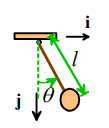1. Introduce variables to describe the motion: We will use the position of the mouse relative to point O (x,y) as the variables.

2. Write down the position vector in terms of these variables:

r = xi + yj

Note that we’ve chosen j to point vertically downwards

3. Differentiate the position vector with respect to time to find the acceleration.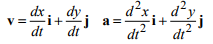4. Draw a free body diagram. We can use the FBD we drew earlier. The force must now be expressed in terms of x and y instead of θ , however. Simple trig shows that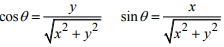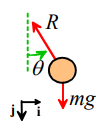The resultant force is therefore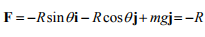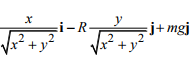5. Write down Newton’s laws of motion. F=ma gives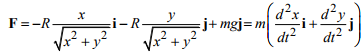This is two equations of motion – we can rearrange them as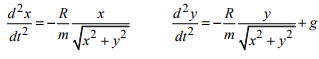6. Eliminate reactions –We could eliminate R if we wanted – but this time we won’t bother. Instead, we will carry R along as an additional unknown, and use MATLAB to calculate it.

7. If there are more unknown variables than equations of motion, look for constraint equations. We now have three unknowns (x,y,R) but only two equations of motion (eliminating R in step 6 won’t help – in this case we will have two unknowns but only one equation of motion). So we need to look for an additional equation somewhere.

The reason we’re missing an equation is that we took x,y to be arbitrary – but of course the mouse has to remain attached to the cable at all times. This means that his distance from O is fixed –i.e.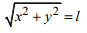This is the missing equation.

We could, if we wanted, use this equation to eliminate one of our unknown variables (doing the algebra by hand). Instead, however, we will use MATLAB to do this for us. For this purpose, we need to turn the equation into a constraint on the accelerations, instead of the position of the particle. To get such an equation, we can differentiate both sides of the constraint with respect to time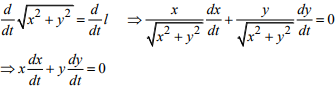This is now a constraint on the velocity. Differentiating again gives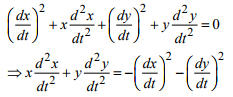Again – if you have trouble doing the derivatives, just use MAPLE (don’t forget to specify that x, y are functions of time, i.e. enter them as x(t), y(t) when typing the constraint formula into MAPLE).

[Aside – when I first coded this problem I tried to constrain the velocity of the particle, instead of the acceleration. This doesn’t work (as I should have known), and produces some rather interesting MATLAB errors – if you are curious, try it and see what happens. If you are even more curious, you might like to think about why you need to constrain accelerations and not velocities.]

7. Identify initial conditions. We know that, at time t=0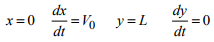8. Solve the equations of motion. We need to (i) reduce the equations to the standard MATLAB form and (ii) write a MATLAB script to solve them.

In the usual way, we introduce vx = dx / dt, v= dy / dt as additional unknowns. Our set of unknown variables is x, y, vx , vy , R. The equations of motion, together with the constraint equation, can be expressed as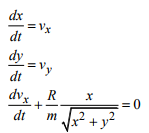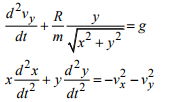This can be expressed as a matrix equation for an unknown vector [dy / dt, R]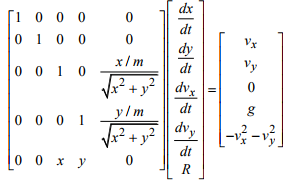We can now use MATLAB to solve the equations for the unknown time derivatives dy / dt , together with the reaction force R. Here’s a MATLAB script that integrates the equations of motion and plots (x,y) as a function of time. Notice that, because we don’t need to integrate R with respect to time, the function ‘eom’ only needs to return dy / dt .

function pendulum

g = 9.81;
l=1;
V0=0.1;
time=20;
w0 = [0,l,V0,0]; % Initial conditions
[t_values,w_values] = ode45(@eom,[0,time],w0);
plot(t_values,w_values(:,1:2));

function dwdt = eom(t,w)
% The vector w contains [x,y,vx,vy]
x=w(1);y=w(2);vx=w(3);vy=w(4);
M = eye(5); % This sets up a 5x5 matrix with 1s on all the diags
M(3,5) = (x/m)/sqrt(x^2+y^2);
M(4,5) = (y/m)/sqrt(x^2+y^2);
M(5,3) = x;
M(5,4) = y;
M(5,5) = 0;
f = [vx;vy;0;g;-vx^2-vy^2];
sol = M\f; % This solves the matrix equation M*[dydt,R]=f for [dwdt,R]
dwdt = sol(1:4); % only need to return time derivatives dw/dt

end
end

Final remarks: In general, it is best to avoid using constraint equations – it makes the problem harder to set up, and harder for MATLAB to solve. Sometimes they are unavoidable, however – in some cases you might not see how to identify a suitable set of independent coordinates; and there are some systems (a rolling wheel is the most common example) for which a set of independent coordinates do not exist. These are given the fancy name `non-holonomic systems’ – mentioning that you happen to be an expert on non-holonomic systems during a romantic candle-light dinner is sure to impress your dates.

3.3.4 Case Study - Simple model of a vehicle

As a representative application of the methods outlined in the preceding section, we will set up and solve the equations of motion for a very simple idealization of a vehicle. This happens to be an example of a non-holonomic system (sorry we aren’t at a romantic dinner). Don’t worry if the model looks rather scary – this calculation is more advanced than anything you would be expected to do at this stage of your career… Our goal is to illustrate how the method we’ve developed in this section can be applied to a real engineering system of interest. You should be able to follow and understand the procedure.

The figure shows how the vehicle is idealized. Here are a few remarks: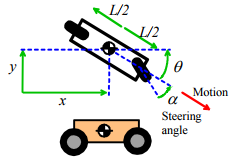1. We consider only 2D planar motion of the vehicle

2. For simplicity we assume the vehicle has only two wheels, one at the front and one at the rear. (It’s not a motorcycle, however, because we won’t account for the vehicle leaning around corners)
3. The car is idealized as a particle with mass m supported on a massless chassis with wheelbase L.
4. Aerodynamic drag forces are assumed to act directly on the particle.
5. The most complicated and important part of a vehicle dynamics model is the description of how the wheels interact with the road. Here, we will just assume that
a. The front and rear of the vehicle have to move in a direction perpendicular to each wheel’s axle. Reaction forces must act on each wheel parallel to the axle in order to enforce this constraint (see the FBD).
b. In addition, we assume that the vehicle has rear-wheel drive. This is modeled as a prescribed force P(t) exerted by the ground on the rear wheel, acting parallel to the rolling direction of the wheel (see the FBD).
c. The front wheel is assumed to roll freely and have negligible mass – this means that the contact force acting on the wheel must be perpendicular to its rolling direction (see the FBD). The vehicle is steered by rotating the front wheel through an angle α(t) with respect to the chassis.

This is a vastly over-simplified model of wheel/road interaction – for example, it predicts that the car can never skid. If you are interested in extending this calculation to a more realistic model, ask us… We’d be happy to give you better equations!

1. Introduce variables to describe the motion: We will use the (x,y) coordinates of car and its orientation θ as the variables.

2. Write down the position vector in terms of these variables: r = xi + yj

3. Differentiate the position vector with respect to time to find the acceleration.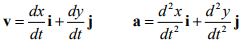4. Draw a free body diagram. The vehicle is subjected to (i) a thrust force P and a lateral reaction force RR acting on the rear wheel, (ii) a lateral reaction force RF acting on the front wheel, and a drag force FD acting at the center of mass.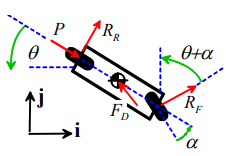The drag force is related to the vehicle’s velocity by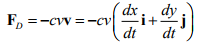where c is a drag coefficient. For a more detailed discussion of drag forces see the first example in this section.

The resultant force on the vehicle is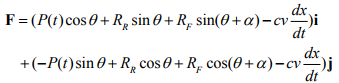Because we are modeling the motion of the vehicle’s chassis, which can rotate, we must also consider the moments acting on the chassis. You should be able to verify for yourself that the resultant moment of all the forces about the particle is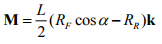5. Write down Newton’s laws of motion. F=ma gives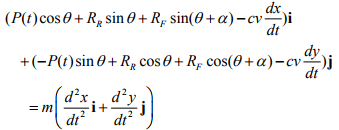This gives two equations of motion; the condition M=0 for the chassis gives one more.

6. Eliminate reactions – It’s easier to eliminate them with MATLAB.

7. If there are more unknown variables than equations of motion, look for constraint equations. This is the hard part of this application. We have two unknown reaction forces, so we need to find two constraint equations that will determine them. These are (i) that the rear of the vehicle must move perpendicular to the axle of the rear wheel; and (ii) the front of the vehicle must move perpendicular to the axle of the front wheel. Consider the rear wheel:

1. Note that the position vector of the rear wheel is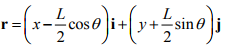2. The velocity follows as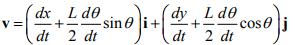3. Note that n = sinθj + cosθj  is a unit vector parallel to the axle of the rear wheel.

4. For the rear of the vehicle to move perpendicular to the rear axle, we must have v.n = 0 . This shows that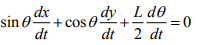Similarly, for the front wheel, we can show that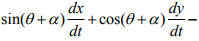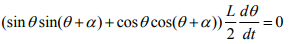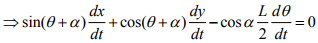where we have used the trig formula cos(A - B) = cos A cos B + sin A sin B .

We need to re-write these equations as constraints on the acceleration of the vehicle. To do this, we differentiate both constraints with respect to time, to see that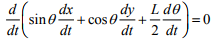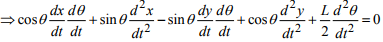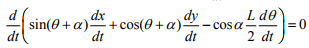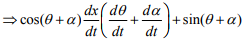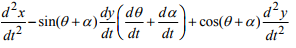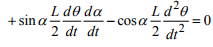7. Identify initial conditions: We will assume that the vehicle starts at rest, with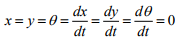7. Solve the equations of motion. We need to write the equations of motion in a suitable matrix form for MATLAB. We need to eliminate all the second derivatives with respect to time from the equations of motion, by introducing new variables. To do this, we define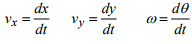as new variables, and then solve for [x, y,θ , vx , vy ,ω] .  We also need to eliminate the unknown reactions. It is not hard to show that the equations of motion, in matrix form, are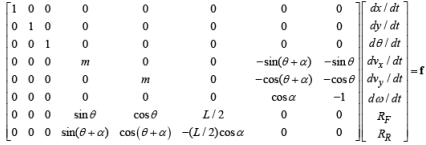where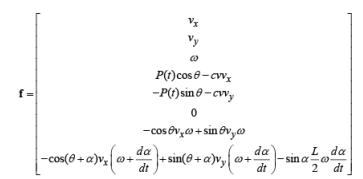Finally, we can type these into MATLAB – here’s a simple script that solves the equations of motion and plots the (x,y) coordinates of the car to show its path, and also plots the speed of the car as a function of time. The example simulates a drunk-driver, who steers with steering angle  α = 0.1+0.2sin(t) , and drives with his or her foot to the floor with P=constant.

function drivemycar

L=1;
m=1;
c=0.1;
time=120;
y0 = [0,0,0,0,0,0];
options = odeset('RelTol',0.00001);
[t_vals,w_vals] = ode45(@eom,[0,time],y0,options);
plot(w_vals(:,1),w_vals(:,2));

% This function behaves like the driver of the car -
% at time t it returns the steering angle alpha % dalpha/dt and the driving force P

alpha = 0.1+0.2*sin(t);
P = 0.2;

end

function dwdt = eom(t,w)
% Equations of motion for the vehicle
%

[alpha,dadt,P] = driver(t); % Find out what the driver is doing
M = zeros(8); % This sets up a 8x8 matrix full of zeros
M(1,1) = 1;
M(2,2) = 1;
M(3,3) = 1;
M(4,4) = m;
M(4,7)=-sin(w(3)+alpha);
M(4,8)=-sin(w(3));
M(5,5)=m;
M(5,7)=-cos(w(3)+alpha);
M(5,8)=-cos(w(3));
M(6,7)=cos(alpha);
M(6,8)=-1;
M(7,4)=sin(w(3));
M(7,5)=cos(w(3));
M(7,6)=L/2;
M(8,4)=sin(w(3)+alpha);
M(8,5)=cos(w(3)+alpha);
M(8,6)=-L*cos(alpha)/2;
v = sqrt(w(4)^2+w(5)^2);
f = [w(4);
w(5);
w(6);
P*cos(w(3))-c*v*w(4);
-P*sin(w(3))-c*v*w(5);
0;
(-cos(w(3))*w(4)+sin(w(3))*w(5))*w(6);
sol = M\f; % This solves the matrix equation M*[dwdt,R]=f for [dwdt,R]
dwdt = sol(1:6); % only need to return time derivatives dw/dt
end
end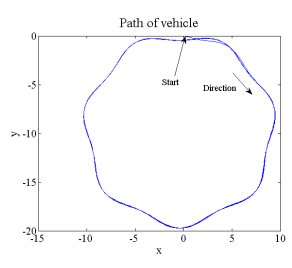Offer running on EduRev: Apply code STAYHOME200 to get INR 200 off on our premium plan EduRev Infinity!

## Introduction to Dynamics and Vibrations- Notes, Videos, MCQs

20 videos|53 docs

,

,

,

,

,

,

,

,

,

,

,

,

,

,

,

,

,

,

,

,

,

;